Tapping into mathematics

Start this free course now. Just create an account and sign in. Enrol and complete the course for a free statement of participation or digital badge if available.

Free course

# 7.2 Square roots

Earlier you met the square function and on most calculators the square root is the second function on the same key. Look to see if this is the case for your calculator and check the calculator handbook on how to use this function. In many cases you will need to press the square root key before the number, instead of afterwards, as for the square key. This is the case on the TI-84. Check that you can find the square root of 25 and of 0.49 (you should get 5 and .7 respectively).

Now find the square root of the number 10 and then square it. (You should get 3.16227766 and then when you square it gives 10 again.) Try squaring a positive number and then square rooting your answer. You should get back to your original number. Like multiplying and dividing, squaring and squarerooting are each other's inverses. If you start with a positive number, square root it and square the answer you get back to the number you started with. The same thing happens if you square the number and then square root it.

Diagrams are often useful in mathematics to illustrate relationships. Figure 3 shows one way of looking at the relationship between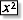and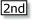[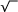]. Starting from a number, 5 in this case, follow the arrows via thebox to the result 25. You can think of this as the ‘doing’ path. Now for the return journey, which can be described as the ‘undoing’ path. Starting from 25 you are led via its square root,[] and you return to the same starting point once more.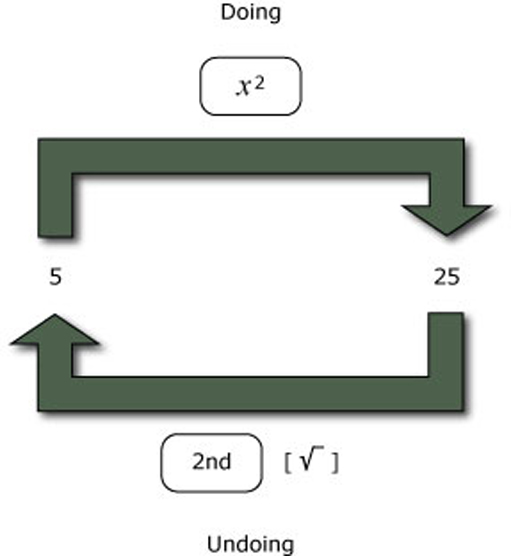Figure 3 Doing and undoing

This notation that mathematical operations often come in pairs, one doing something to a number and the other undoing it, occurs again and again in mathematics. Try to get the feel of the idea and be on the lookout for other pairs of mathematical operations that can be thought about in terms of doing and undoing. What other mathematical operations have you come across that can be described by a ‘doing–undoing’ diagram?

Addition and subtraction are inverses of each other, as are multiplication and division, and you may have thought of some others. Figure 4 shows the doing–undoing diagram for the operations of adding and subtracting 3 from a number (any number – choose one and try it). Notice that you can start the cycle at either side.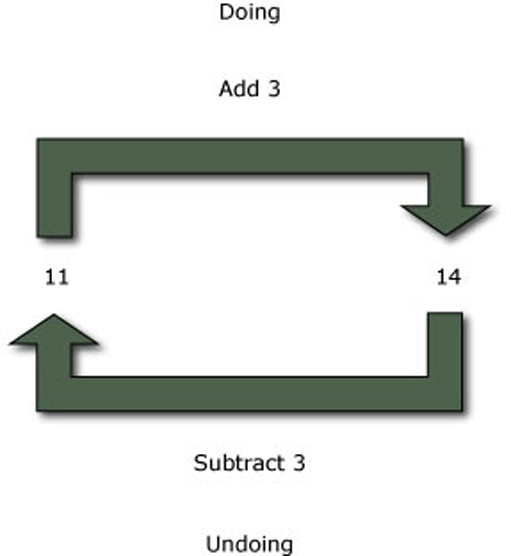Figure 4 Adding and subtracting 3

Similarly, Figure 5 shows the diagram for multiplying and dividing any number by 5. In each case, you can see that what one operation does, its companion inverse undoes, and again you can start with either operation.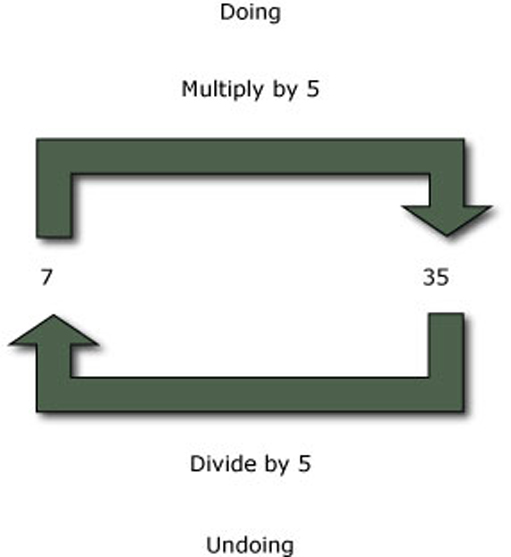Figure 5 Multiplying and dividing by 5

The earlier example of square and square root being inverses of each other seems to work either way round – if you start with a positive number, square it and then find the square root of the result, you get back to where you started, as in Figure 3. Similarly if you try it the other way round, as in Figure 6, by starting with a number, finding its square root and then squaring it you must also get back to the number you started with, surely?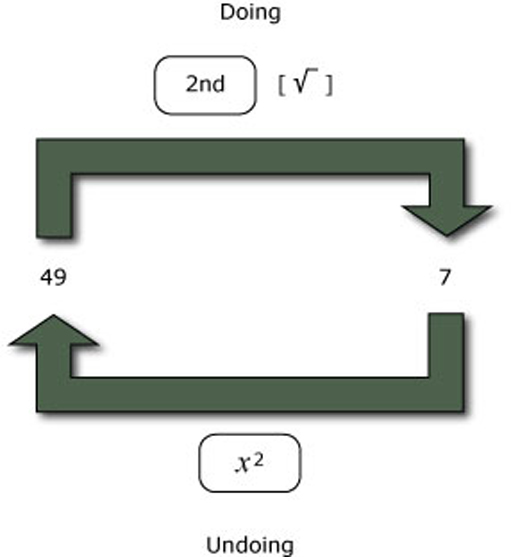Figure 6 Square rooting and squaring

Well no, not always! It turns out that this doing–undoing cycle works only if you start with a positive number. It runs into trouble if you start with a negative number. There are two problems and which one occurs depends on which operation you try first. If you start with, say, 8 and use the calculator to square it (remember that you need to use brackets here!), you get the positive number 64. Now find the square root of 64 and your calculator will display 8 rather than 8, as shown in Figure 7.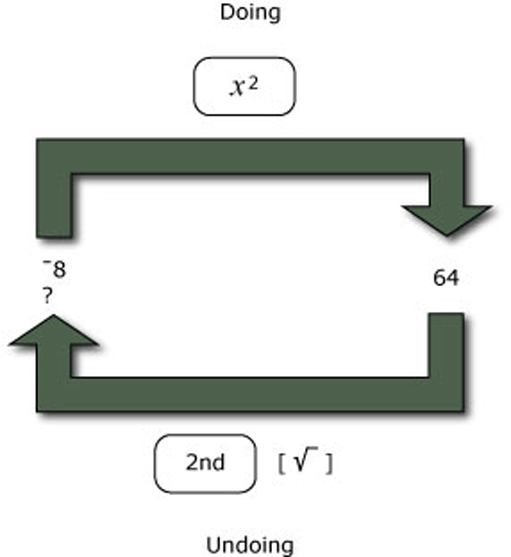Figure 7 Squaring a negative number and then finding the square root

Why is this?

Think for a moment about what happens when a positive number, say 8, is squared. Squaring 8 gives 8 × 8 = 64; another positive number. Finding the square root takes you back to the starting number 8.

Now consider what happens if you start with a negative number and square it. For example, when8 is squared, the result is 8 × 8 = 64. Now, when you try to return to the start by finding the square root of 64 there is no means of knowing, by looking at the 64 on its own, whether you should get back to 8 or +8.

When you use the calculator to find the square root of a positive number you need to be aware of this ambiguity. The calculator gives only the positive answer; you must decide whether it is the positive or negative value that is appropriate.

MU120_4MCAL